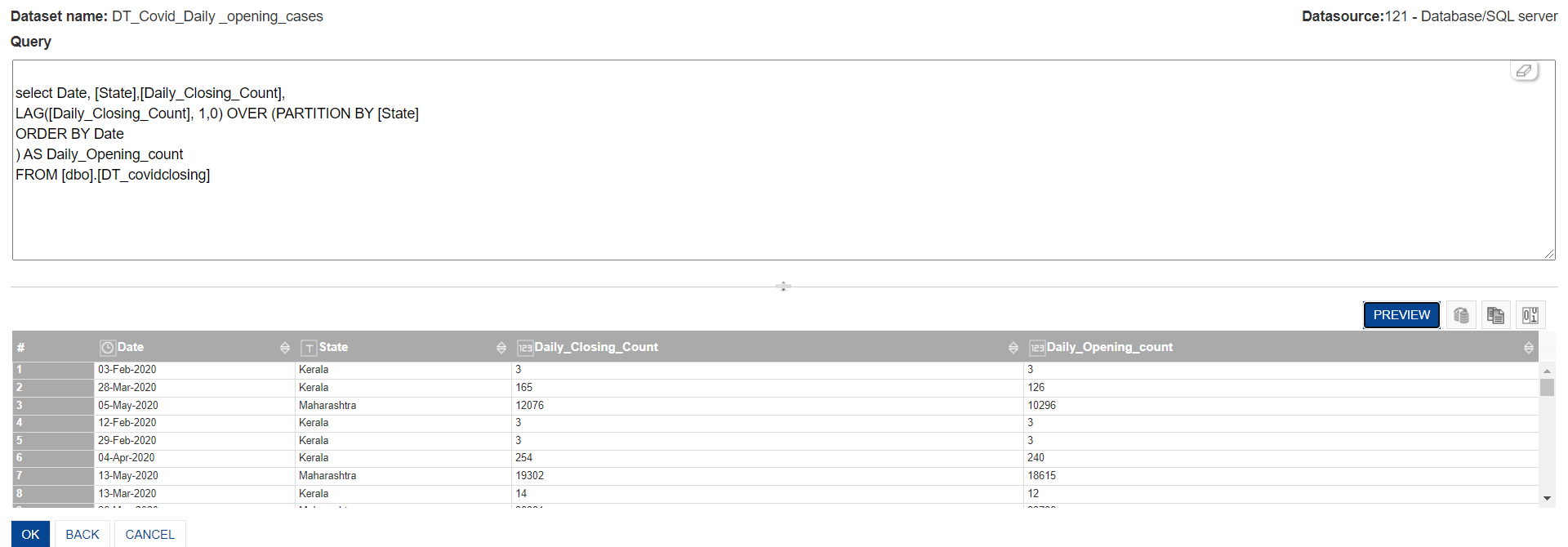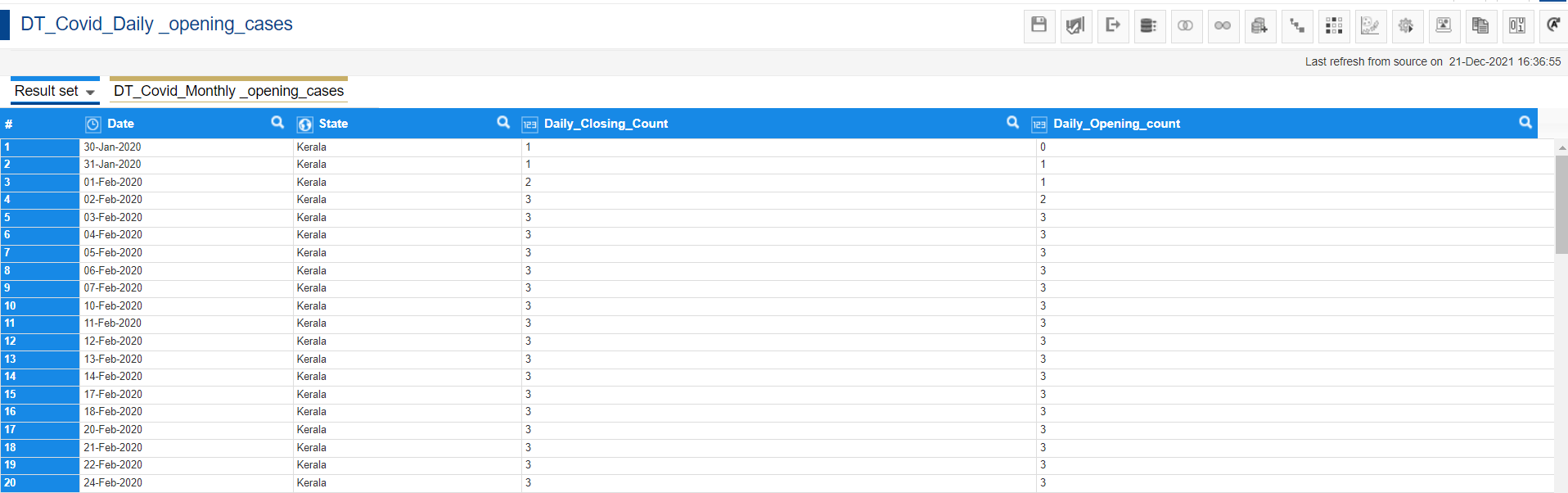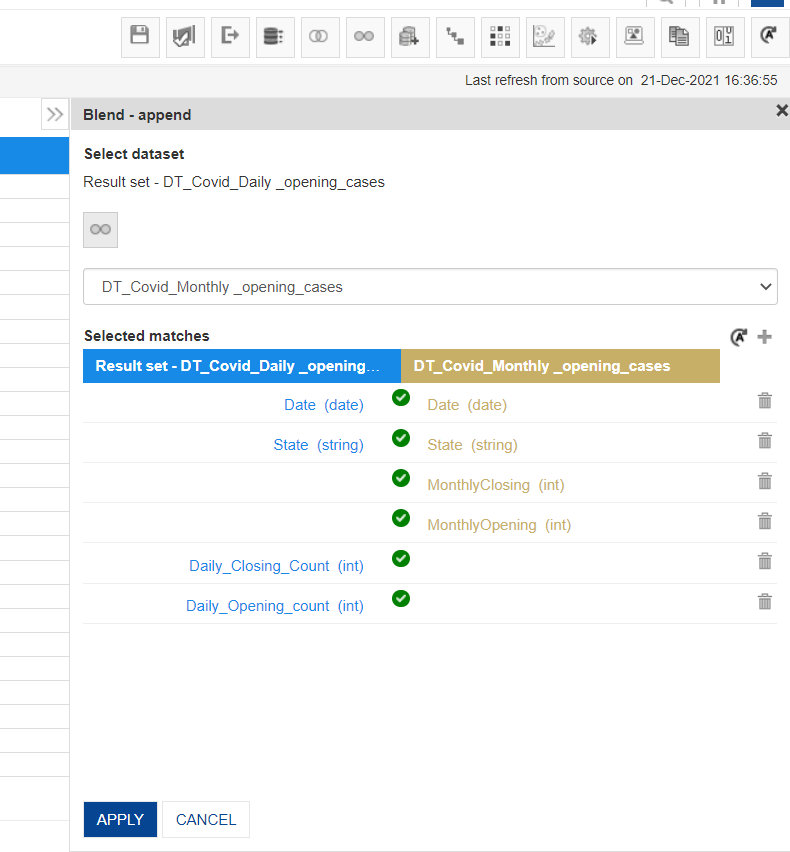Scenario:

The data has the state wise daily closing count (cumulative count) of covid cases.

Requirement:

Derive the daily opening and monthly opening covid count for each state.Solution:

There are 3 datasets created in achieving the scenario.

1. Daily closing count dataset (Base Dataset): This dataset contains the daily closing counts with respect to Daily dates against the state.
2.  Daily closing and opening count dataset (Through Daily Query): This dataset contains the daily closing counts and its opening count with respect to Daily dates against the state.
3.  Monthly closing and opening count dataset (Through Monthly Query): This dataset has the monthly opening and closing counts of particular state and its respective month.

Daily

For Daily Opening and Closing Count

1. Sorting the dataset (Daily closing count dataset) - Sort the data in ascending order first by date and then by state to get the number of daily closing count in an appropriate sequence.

2. Export to SQL Server (Daily closing count dataset) - Now Export the dataset to SQL server with the help of which we will be deriving the daily opening cases. As the closing of any particular date will become the opening of its next date. For example: If 30 was the closing count of 15th January, then this 30 will act as the opening count for 16th January.

3. Deriving Daily Opening Count through SQL Query - With the help of SQL query which we would execute on SQL server we would derive the daily opening count of respective state.

The query used for deriving Daily Opening counts is as follows (in SQL Server):

select Date, [State],[Daily_Closing_Count],

LAG([Daily_Closing_Count], 1,0) OVER (PARTITION BY [State]

ORDER BY Date

) AS Daily_Opening_count

FROM [dbo].[DT_covidclosing]

Here we are taking columns “Date”, “state” and “[Daily_Closing_Count]” then using a LAG function which is used to get a value from previous row on column [Daily_Closing_Count] as we know closing count of today will be the opening of tomorrow and then we are partitioning it by state ( partition by -used to part the rows of table into groups) as the closing count of each state differs and then we have order it by Date so it will arrange in proper sequence, the column so derived we have name it as “Daily_Opening_count” from table FROM [dbo].[DT_covidclosing].

Below is the resultant output that we get with the above query: -Note :- The functions used in the query are of SQL Server. This query syntax may differ in other database systems.

4. Create the new Dataset with SQL Server as Data source (Daily closing and opening count dataset) -  Create a new dataset in smarten with the above query. Now this dataset has state wise daily closing counts with its daily opening counts.

Monthly

For Monthly Opening and Closing Count

1. Deriving Monthly Opening and Closing Count through SQL Server -  With the help of Daily closing count we will derive the Monthly closing state wise. Run a query to find monthly opening and closing count.

Below is the Query for deriving the Monthly opening and closing Count:

The monthly counts are achieved basically by rolling up the daily counts with the help of sub-queries which are defined below.

select  c.*,lag(c.[MonthlyClosing ],1,0) over (partition by c.state order by c.dt)      as MonthlyOpening from

(select a.State, a.dt, b.[Daily_Closing_Count] as MonthlyClosing from

(select max(Date) as dt,[State],month(Date) as monthname, year(Date) as  yearname

from [dbo].[DT_covidclosing]

group by [State],month(Date) , year(Date)) a

Left join

(select Date,[State],[Daily_Closing_Count]

from [dbo].[DT_covidclosing]) b

on a.dt=b.Date and a.state=b.state) c

Here we are using subqueries.

• First we calculated max(Date) as dt,[State],month(Date) as monthname, year(Date) as yearname from [DT_covidclosing] with which we got the state wise count of the maximum date of that month then we have used group by function on state ,month(Date) , year(Date) column and name it as ‘a’.

• Then we applied a left join joining Table ‘a’ with Table ‘b’. In Table ‘b’ we have calculated Date, [State], [Daily_Closing_Count] from table [DT_covidclosing]) on a.dt=b.Date and a.state=b.state and name it as ‘c’.

• Then we have calculated a.State, a.dt, b.[Daily_Closing_Count] as MonthlyClosing from above subquery.

• In last we have calculated c.*, lag(c.[MonthlyClosing ],1,0) over (partition by c.state order by c.dt) as MonthlyOpening from above subquery.

Below is the resultant output that we get with the above query:-2. Create the new Dataset with SQL Server as Data source (Monthly closing and opening count dataset)  Create a new dataset in smarten which has monthly opening and closing count.

3. Append the Monthly Dataset with Daily Dataset (Daily closing and opening count dataset) - append monthly daily opening and closing count in daily opening and closing dataset on date and state column. This will give us daily and monthly count of covid cases.

Below are the steps to achieve it in smarten.

For Daily opening and closing count

Step 1: Sorting the dataset (Daily closing count dataset)

To begin, first we need to sort the Date and state in ascending order so that we get the data on a daily basis.

Right click on Date and the state -> Sort -> AscendingStep 2: Export to SQL Server (Daily closing count dataset)

Now ingest this data which is date and state wise Daily Closing to the SQL Server from Smarten SSDP.
For that Go to Publish -> To Other Data source -> Choose the other data source as SQL Server Database -> Data source name -> Table nameStep 3: Deriving Daily Opening Count through SQL Query

Now with the help of Sql query we will find out the Daily Opening count against its Daily Closing Count State and Date wise.

Below is the query to find the Daily Opening Count

select Date, [State],[Daily_Closing_Count],

LAG([Daily_Closing_Count], 1,0) OVER (PARTITION BY [State]

ORDER BY Date

) AS Daily_Opening_count

FROM [dbo].[DT_covidclosing]

Here we are taking columns “Date”, “state” and “[Daily_Closing_Count]” then using a LAG function which is used to get a value from previous row on column [Daily_Closing_Count] as we know closing count of today will be the opening of tomorrow and then we are partitioning it by state ( partition by -used to part the rows of table into groups) as the closing count of each state differs and then we have order it by Date so it will arrange in proper sequence, the column so derived we have name it as “Daily_Opening_count” from table FROM [dbo].[DT_covidclosing].

Step 4: Create the new Dataset with SQL Server as Data source (Daily closing and opening count dataset)

Now the data so obtained make the new dataset in Smarten with data source as the SQL Server

For that New -> Dataset -> Name the Dataset -> Choose the Data source -> Enable Paste ready to use query -> Click on nextNow paste the above query in the query window as shown below, then preview it and then go ahead with Clicking on Ok.Below is the resultant dataset that we get with the query.For Monthly Opening and Closing Count

Step 1: Deriving Monthly Opening and Closing Count through SQL Server

With the help of Daily closing count we would find the Monthly opening and closing count with respect to the month end date and its state.

Below is the query to find the Monthly Opening and Closing Count

select  c.*,lag(c.[MonthlyClosing ],1,0) over (partition by c.state order by c.dt) as MonthlyOpening from

(select a.State, a.dt, b.[Daily_Closing_Count] as MonthlyClosing from

(select max(Date) as dt,[State],month(Date) as monthname, year(Date) as yearname

from [dbo].[DT_covidclosing]

group by [State],month(Date) , year(Date)) a

Left join

(select Date,[State],[Daily_Closing_Count]

from [dbo].[DT_covidclosing]) b

on a.dt=b.Date and a.state=b.state) c

Here we are using subqueries .

• First we calculated max(Date) as dt,[State],month(Date) as monthname, year(Date) as yearname from [DT_covidclosing] with which we got the state wise count of the maximum date of that month then we have used group by function on state ,month(Date) , year(Date) column and name it as ‘a’.

• Then we applied a left join joining Table ‘a’ with Table ‘b’. In Table ‘b’ we have calculated Date, [State], [Daily_Closing_Count] from  table [DT_covidclosing]) on  a.dt=b.Date and a.state=b.state and name it as ‘c’.

• Then we have calculated a.State, a.dt, b.[Daily_Closing_Count] as MonthlyClosing from above subquery.

• In last we have calculated c.*,lag(c.[MonthlyClosing ],1,0) over (partition by c.state order by c.dt) as MonthlyOpening from above subquery

Step 2: Create the new Dataset with SQL Server as Data source (Monthly closing and opening count dataset)

Create a new dataset for Monthly Opening and Closing Count in Smarten with data source as the SQL Server.Step 3: Append the Monthly Dataset with Daily Dataset (Daily closing and opening count dataset)

Now we will append monthly daily opening and closing count in daily opening and closing dataset

Open the Daily Opening and Closing Dataset -> Add the Dataset -> Append

Add the Monthly dataset from ‘Add datasets’ option in the toolbar. The Monthly dataset will be added in a new tab.To append the dataset, click on the Blend-Append button on the tool bar.

Then map the columns of both the Datasets.

The mapping column are:

1. Date = Date
2. State = StateHence the Monthly Dataset will be appended below the Daily Counts.

For Example - Filter State = Kerala and Date = Jan-2020, then below is the resultant data that we would get.